Coulomb's law facts for kids

Kids Encyclopedia Facts
(Redirected from Coulomb force)

Coulomb's law is a function developed in the 1780s by physicist Charles Augustin de Coulomb. It explains how strong the force will be between two electrostatic charges. Electrostatic means electric charges without any motion.

Direction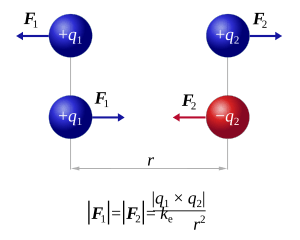This picture shows how Coulomb's Force act; similar charges pushing against each other and opposite charges attract each other

Let's think of two electric charges existing in an empty space. If the two charges are opposite, (+) and (-) charges for example, they will attract each other. And if two charges are both the same, both (+) or both (-) for example, they will push each other. This is similar to how magnets act, as N and S attract each other, and as N and N, S and S push each other.

This is because electric charges make an electric field. If two fields exist in the same space at the same time, then the two fields exert (~ put) force on each other. The force they make on each other is called Coulomb's force or electrostatic force. Coulomb's law explains how big the force will be.

Scale

Coulomb's law explains the scale between two electric charges. The scale of electrostatic force follows the function below.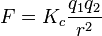$F = {K_c}\frac{q_1q_2}{r^2}$

Coulomb's law explains that the force scale F is relative to ratio of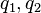$q_1,q_2$,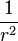$\frac{1}{r^2}$.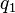$q_1$ and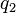$q_2$ are the scales of each electric charge.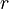$r$ is the distance between the two electric charges. And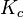$K_c$ has a certain value. It does not change relative to$q_1$,$q_2$ or$r$. While${K_c}$ remains constant, when multiples of$q_1$ and$q_2$ become bigger, the electrostatic force will also get bigger. When the distance$r$ become bigger, the electrostatic force will become smaller to ratio of$\frac{1}{r^2}$.
The exact size of$K_c$ is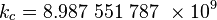\begin{align} k_c &= 8.987\ 551\ 787\ \times 10^9 \\ \end{align}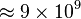$\approx 9 \times 10^9$N m2 C−2 (or m F−1). This constant is called Coulomb's Force Constant or Electrostatic Force Constant.

Inverse-square law

The relation between the force of pushing or pulling (F) and the distance between the particles ($r$) follows the Inverse-square Law. Inverse-square law means that when the distance$r$ grows bigger, the force gets weaker by the ratio$\frac{1}{r^2}$. Gravitation, Electromagnetic radiation, Sound Intensity also follows this law.

Images for kidsCoulomb's law Facts for Kids. Kiddle Encyclopedia.